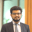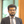Trusted answers to developer questions
Trusted Answers to Developer Questions

Related Tags

animation
javascript

# How to create a custom easing in anime.jsRana Abdul Muneem

Grokking Modern System Design Interview for Engineers & Managers

Ace your System Design Interview and take your career to the next level. Learn to handle the design of applications like Netflix, Quora, Facebook, Uber, and many more in a 45-min interview. Learn the RESHADED framework for architecting web-scale applications by determining requirements, constraints, and assumptions before diving into a step-by-step design process.

## What are easings?

Easings are mathematical functions that define the rate of change in animation progress. Anime.js provides many in-built easings, but we can also define custom easings.

### Syntax

Custom easings are made as a function-based value. We return another function that takes time as a parameter within the function-based value.

Note: An understanding of function-based values is necessary to understand custom easings. Function-based values and their parameters: targets, index, and length are explained here.

The syntax is as follows:

anime({
...,
easing: function (target, index, length) {
return function (time) {
return ...;
};
}
});


### Parameters

The time parameter represents the current time of the animation. This parameter ranges from $0$ to $1$.

The value returned by the nested function is the progress of the animation ranging from $0$ to $1$.

Generally, we define an easing function $E$ such that:

In other words, at time $t=0$, the animation would be at $0$ progress; at $t=1$, the animation would be at $1$ or complete progress.

### Example

The example below shows how to create a custom easing function. We make a 'dribbling' effect by using the trigonometric function given below:

This equation generates the following progress vs. time graph:

### Code

The code below describes how to use this formula as an easing:

Using a custom formula as an easing

#### Explanation

In the HTML section, we create a box using a div element and a box class. In the JavaScript section, we create the animation using the anime() function.

• Line 2: We target the box using its box class.
• Lines 3–5: We set parameters for the animation that controls the box's horizontal movement and timing. The tranlsateX property moves the box right to 500px, the duration is set to 1000 milliseconds, and the delay before the start of the animation is set to 500 milliseconds.
• Lines 6–10: We set the easing parameter to a function-based value. Inside this function-based value, we return a function that takes time as a parameter. This nested function returns the trigonometric function that we have defined above.

RELATED TAGS

animation
javascript

CONTRIBUTORRana Abdul Muneem